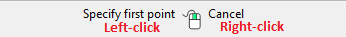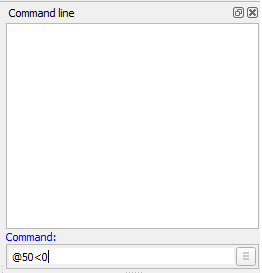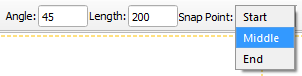* Home

usage:animated

# Animated Tutorials

In this Animated Tutorials series, one will get a basic ideas of - how tools can be used to create drawings.

This tutorial will guide step by step to understand from very basic behavior of tools. In other word, it can be an Animated User Manual.

Contents of this Tutorial will follow as mentioned below.

• Line
• Circle
• Modify

Before moving to tutorials, let's see few basics of - how command-line, mouse, and Tool-option used as input.

#### (1)

`MOUSE` (located at status bar)When command is changed, one will see differences such as

• specify first point
• specify position
• and so on…
• `Right-click` works as same as Esc, To exit any commands, Right-click two times escapes the command.

#### (2)

`Command-line`Command-line is used for inputs such as Length, Angle, Points, and can be input the same after the particular command is activated.

#### (3)

`Tool-option`

Different tools shows the different option, check-it out by various different tools such as Line, Angle, Circle, Rectangle, etc.## Contents Of Tools

Line Circle Modify
2 points Center Point Move / Copy
Angle 2 Points Rotate
Vertical 3 Points Mirror
Rectangle Center, Radius Move and Rotate
Parallel through points Tangential 2 Circles, 1 Point Revert Direction
Parallel Tangential, 2 Point Trim
Bisector Tangential 2 Circles, Radius Trim Two
Tangent (P,C) Tangential 3 Circles Lengthen
Tangent (C,C) Offset
Tangent Orthogonal Bevel
Orthogonal Fillet
Relative Angle Divide
Polygon (Cen, Cor) Stretch
Polygon (Cen, Tan) Properties
Polygon (Cor, Cor) Attributes
Explode text
Explode
Delete Selected